Value of a polynomial at a given point

Chapter 2 Class 9 Polynomials
Concept wise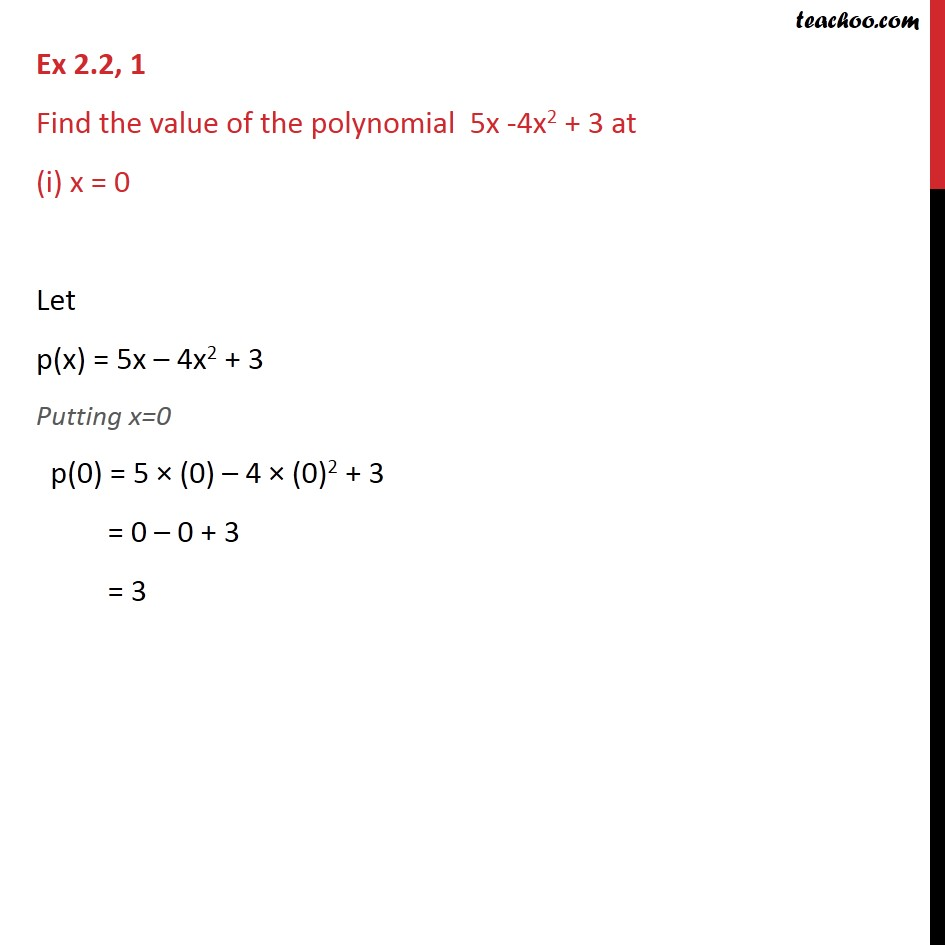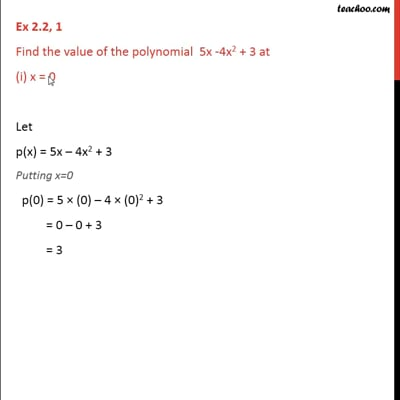This video is only available for Teachoo black users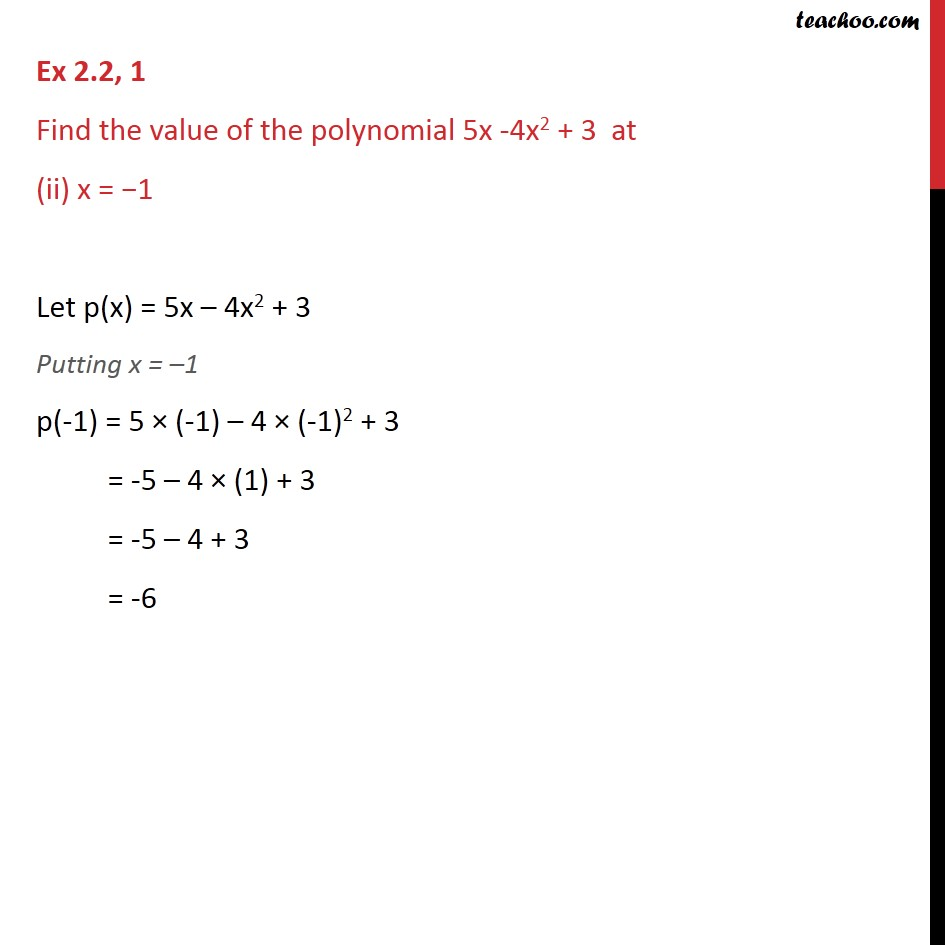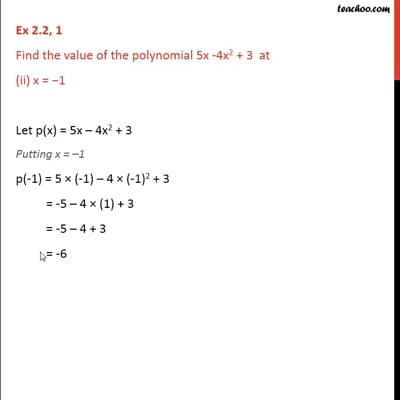This video is only available for Teachoo black users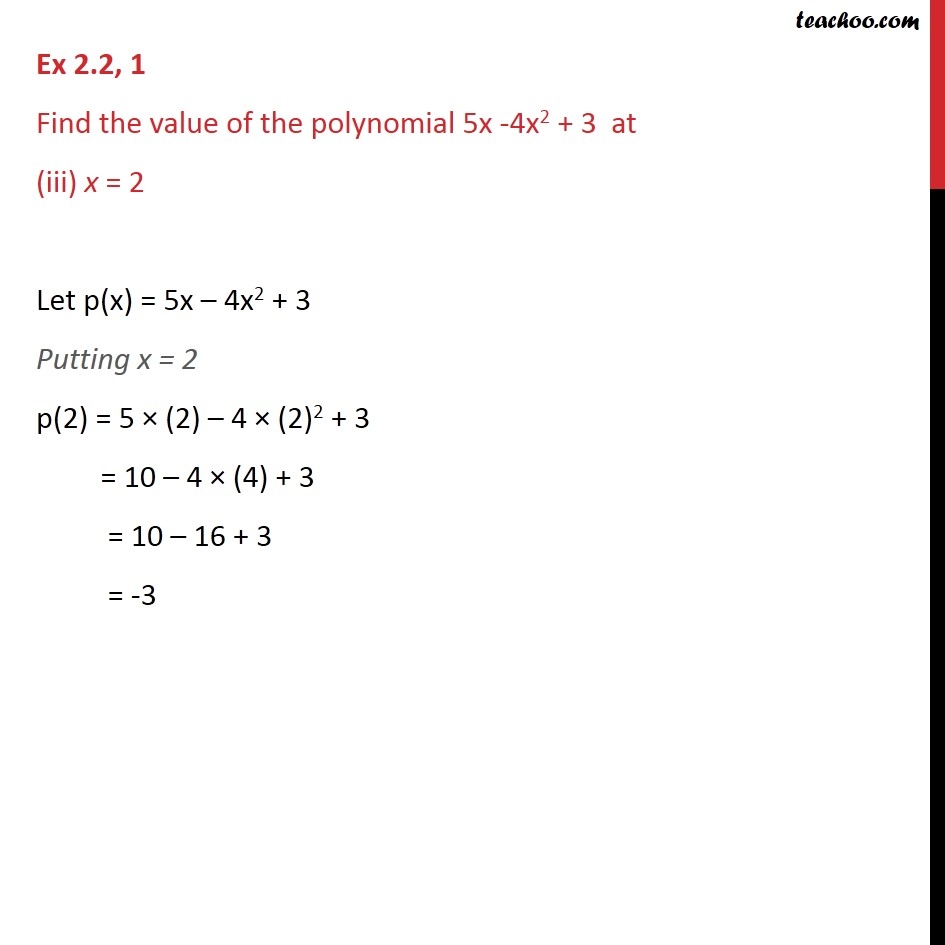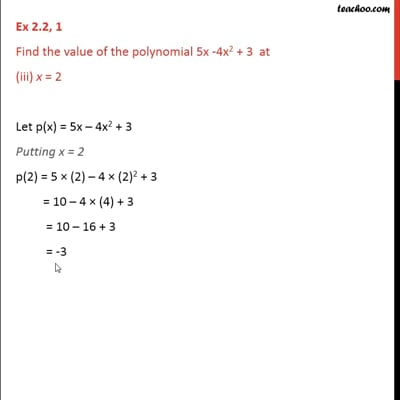This video is only available for Teachoo black users

Solve all your doubts with Teachoo Black (new monthly pack available now!)

### Transcript

Ex 2.2, 1 Find the value of the polynomial 5x -4x2 + 3 at (i) x = 0 Let p(x) = 5x – 4x2 + 3 Putting x=0 p(0) = 5 × (0) – 4 × (0)2 + 3 = 0 – 0 + 3 = 3 Ex 2.2, 1 Find the value of the polynomial 5x -4x2 + 3 at (ii) x = −1 Let p(x) = 5x – 4x2 + 3 Putting x = –1 p(-1) = 5 × (-1) – 4 × (-1)2 + 3 = -5 – 4 × (1) + 3 = -5 – 4 + 3 = -6 Ex 2.2, 1 Find the value of the polynomial 5x -4x2 + 3 at (iii) x = 2 Let p(x) = 5x – 4x2 + 3 Putting x = 2 p(2) = 5 × (2) – 4 × (2)2 + 3 = 10 – 4 × (4) + 3 = 10 – 16 + 3 = -3